# Motor Notes : Method of Calculating the Power Consumption of a Brushed Motor Driver: Part 2

2022.09.07

In succession to the previous article, we calculate the power consumption of a brushed motor driver. In the last article, a representative method of constant-voltage driving of a brushed motor was explained; here we explain how to calculate power consumption for PWM driving, which is another representative driving method.

## Method of Calculating the Power Consumption of a Brushed Motor Driver

②Equations to calculate power consumption for PWM driving

We begin by indicating the operating equivalent circuit under PWM driving and the operating voltage and current waveforms. The operating equivalent circuit on the left is an example of the MOSFET states when a voltage is applied, and that on the right indicates the MOSFET states for current regeneration (MOSFETs that do not affect operation are omitted).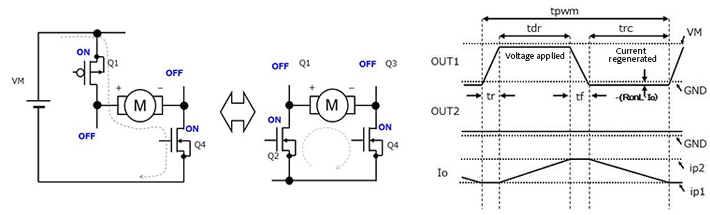Operation in one PWM period (tpwm) is, at the output OUT1, divided into the following four parts.

• ・tr: Time of the transition from low to high
• ・tdr: Time during which high is maintained and current is supplied
• ・tf: Time of the transition from high to low
• ・trc: Time during which low is maintained and current is regenerated

On the other hand, the output OUT2 is always low.

To simplify matters, we have assumed that in these operations, when the output transitions from high to low or from low to high the voltage change is a straight line, and the current is constant and unchanging. Moreover, the current change during voltage application and current regeneration is also linear (see the above waveforms).

The energy consumed in the parts where the voltage changes is obtained by integrating the product of a time function for the voltage and a time function for the current over each of the time periods. The power consumption in the parts where the current changes can be obtained by integrating the product of the resistance and the square of a time function of the current over each of the time periods.

Energy consumed in the tr part: Er is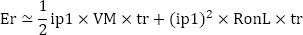Energy consumed in the tf part: Ef is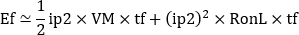Energy consumed in the tdr part: Edr is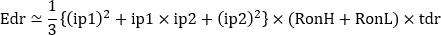Energy consumed in the trc part: Erc is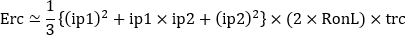The output power consumption Pc_ot is found by dividing the sum of these four parts by the total time.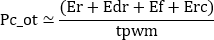Upon adding to this the product of the power supply voltage Vcc and the circuit current Icc supplied by the power supply other than the output current, the overall power consumption Pc is obtained.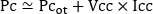When tr and tf are sufficiently small compared with tpwm, and there is almost no change in current so that ip1＝ip2, the power consumption becomes as follows.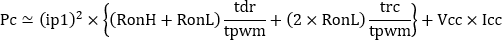In other words, it is the sum of the ratio of the driving time and the regeneration time to the PWM cycle time of the power generated by the current path that changes between driving and regenerating.

In PWM driving, instead of a constant voltage being applied as in constant-voltage driving, pulses are applied, so that calculation equations become somewhat complicated; the example explained here should help the reader understand how to approach the problem.